## Example Questions

### Example Question #1 : Sequences

How many integers in the following infinite series are positive: 100, 91, 82, 73 . . . ?

9

13

11

12

10

12

Explanation:

The difference between each number in the series is 9. You can substract nine 11 times from 100 to get 1: 100 – 9x11 = 1. Counting 100, there are 12 positive numbers in the series.

### Example Question #1 : How To Find The Common Difference In Sequences

In a sequence of numbers, each term istimes larger than the one before it. If the 3rd term of the sequence is 12, and the 6th term is 96, what is the sum of all of the terms less than 250?

372

381

378

192

384

381

Explanation:

Let's call the first term in the sequence a1 and the nth term an

We are told that each term is r times larger than the one before it. Thus, we can find the next term in the sequence by multiplying by r.

a1 = a1

a2 = r(a1)

a3 = r(a2) = r(r(a1)) = r2(a1)

a4 = r(a3) = r(r2(a1)) = r3(a1)

an = r(n–1)a1

We can use this information to find r.

The problem gives us the value of the third and the sixth terms.

a3 = r2(a1) = 12

a6 = r5(a1) = 96

Let's solve for a1 in terms of r and a3

a1 = 12/(r2)

Let's then solve for a1 in terms of r and a6.

a1 = 96/(r5)

Now, we can set both values equal and solve for r.

12/(r2) = 96/(r5)

Multiply both sides by r5 to get rid of the fraction.

12r5/r2 = 96

Apply the property of exponents which states that ab/ac = ab–c.

12r3 = 96

Divide by 12 on both sides.

r3 = 8

Take the cube root of both sides.

r = 2

This means that each term is two times larger than the one before it, or that each term is one half as large as the one after it.

a2 must equal a3 divided by 2, which equals 12/2 = 6.

a1 must equal a2 divided by 2, which equals 6/2 = 3.

Here are the first eight terms of the sequence:

3, 6, 12, 24, 48, 96, 192, 384

The question asks us to find the sum of all the terms less than 250. Only the first seven terms are less than 250. Thus the sum is equal to the following:

sum = 3 + 6 + 12 + 24 + 48 + 96 + 192 = 381

### Example Question #2 : How To Find The Common Difference In Sequences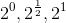Find the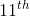term of the sequence above.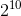Explanation:

The sequence is geometric with a common ratio of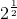.

The formula for finding the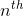term of the sequence is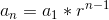.

So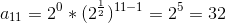### Example Question #3 : How To Find The Common Difference In Sequences

Which of the following are not natural numbers?

I. 1

II. 0

III. 349010

IV. -2

V. 1/4

II, III, IV, V

II, IV, V

I, IV, V

I, V

IV, V

II, IV, V

Explanation:

Natural numbers are defined as whole numbers 1 and above. II, IV, V are not natural numbers.

### Example Question #4 : How To Find The Common Difference In Sequences

An arithmetic sequence begins as follows: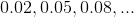Give the first integer in the sequence.The sequence has no integers.Explanation:

Subtract the first term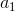from the second term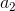to get the common difference: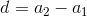Setting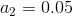and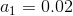,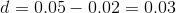Ifis in the sequence, then there is an integersuch that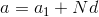, or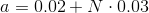Solving for,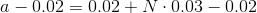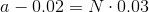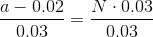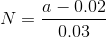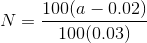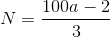Therefore, we seek the least positive integer value ofsuch thatis itself an integer. By trial and error, we see: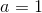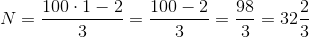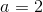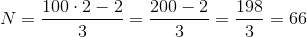,

which is an integer.

Therefore, 2 is the first integer value in the sequence.